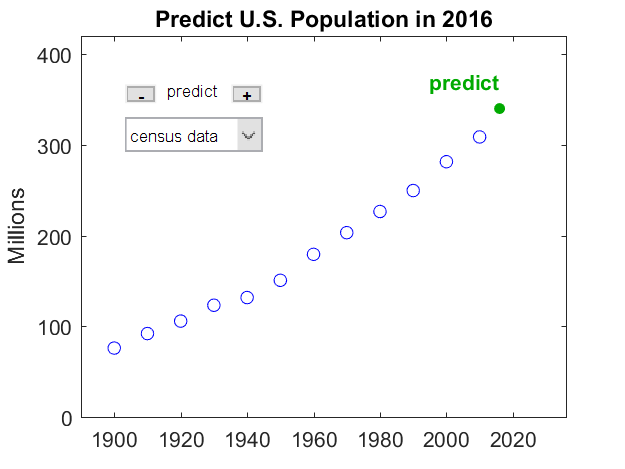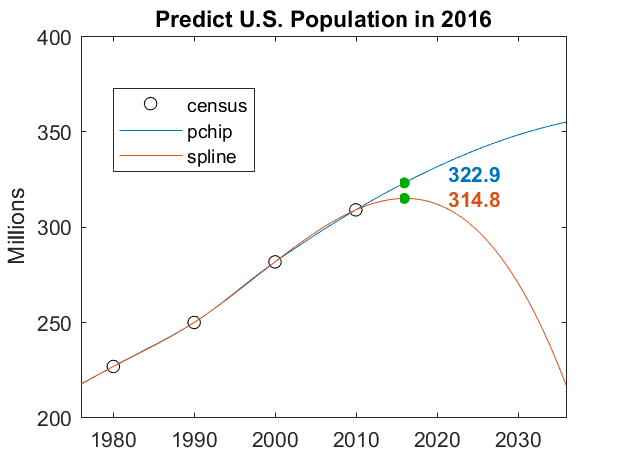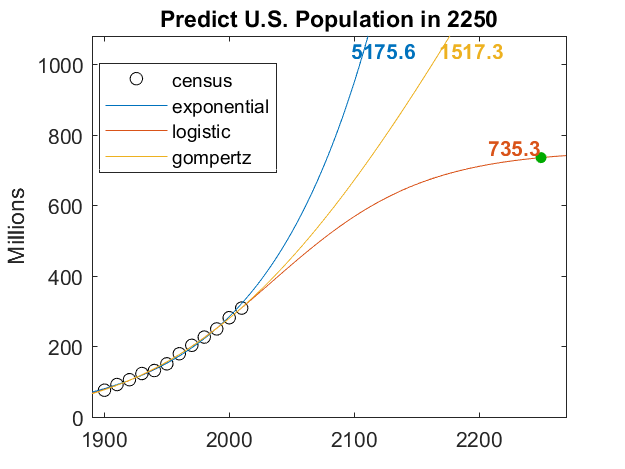# Fitting and Extrapolating US Census Data

A headline in the New York Times at the end of 2016 said "Growth of U.S. Population Is at Slowest Pace Since 1937". This prompted me to revisit an old chestnut about fitting and extrapolating census data. In the process I have added a couple of nonlinear fits, namely the logistic curve and the double exponential Gompertz model.

### Contents

#### Oldie, But Goodie

This experiment is older than MATLAB. It started as an exercise in Computer Methods for Mathematical Computations, by Forsythe, Malcolm and Moler, published by Prentice-Hall 40 years ago. We were using Fortran back then. The data set has been updated every ten years since. Today, MATLAB graphics makes it easier to vary the parameters and see the results, but the underlying mathematical principles are unchanged:

• Using polynomials of even modest degree to predict the future by extrapolating data is a risky business.

Recall that the famous computational scientist Yogi Berra said

• "It's tough to make predictions, especially about the future."

#### Data

The data are from the decennial census of the United States for the years 1900 to 2010. The population is in millions.

   1900    75.995
1910    91.972
1920   105.711
1930   123.203
1940   131.669
1950   150.697
1960   179.323
1970   203.212
1980   226.505
1990   249.633
2000   281.422
2010   308.746

The task is to extrapolate beyond 2010. Let's see how an extrapolation of just six years to 2016 matches the Census Bureau announcement. Before you read any further, pause and make your own guess.

#### App

Here's is the opening screen of the January 2017 edition of my censusapp, which is included in Cleve's Laboratory. The plus and minus buttons change the extrapolation year in the title. If you go beyond 2030, the plot zooms out.#### Models

The pull-down menu offers these models. Forty years ago we had only polynomials.

   models = {'census data','polynomial','pchip','spline', ...
'exponential','logistic','gompertz'}'

models =
7×1 cell array
'census data'
'polynomial'
'pchip'
'spline'
'exponential'
'logistic'
'gompertz'


#### 2016

The Census Bureau news release that prompted the story in The Times said the official population in 2016 was 323.1 million. That was on Census Day, which is April 1 of each year. The Census Bureau also provides a dynamic Population Clock that operates continuously. But let's stick with the 323.1 number.

#### Polynomials

Polynomials like to wiggle. Constrained to match data in a particular interval, they go crazy outside that interval. Today, there are 12 data points. The app lets you vary the polynomial degree between 0 and 11. Polynomials with degree less than 11 approximate the data in a least squares sense. The polynomial of degree 11 interpolates the data exactly. As the degree is increased, the approximation of the data becomes more accurate, but the behavior beyond 2010 (or before 1900) becomes more violent. Here are degrees 2 and 7, 9, 11, superimposed on one plot.

The quadratic fit is the best behaved. When evaluated at year 2016, it misses the target by six million. Of course, there is no reason to believe that the US population grows like a second degree polynomial in time.

The interpolating polynomial of degree 11 tries to escape even before it gets to 2010, and it goes negative late in 2014.#### Splines

MATLAB has two piecewise cubic interpolating polynomials. The classic spline is smooth because it has two continuous derivatives. Its competitor pchip sacrifices a continuous second derivate to preserve shape and avoid overshoots. I blogged about splines and pchips a few years ago.

Neither is intended for extrapolation, but we will do it anyway. Their behavior beyond the interval is determined by their end conditions. The classic spline uses the so-called not-a-knot condition. It is actually a single cubic in the last two subintervals. That cubic is also used for extrapolation beyond the endpoint. pchip uses just the last three data points to create a different shape-preserving cubic for use in the last subinterval and beyond.

Let's zoom in on the two. Both are predicting a decreasing rate of growth beyond 2010, just as the Census Bureau is observing. pchip gets lucky and comes within 0.2 million of the announcement for 2016.#### Three Exponentials

As I said, there is no good reason to model population growth by a polynomial, piecewise or not. But because the rate of growth can be expected to be proportional to the size of the population, there is good reason to use an exponential.

$$p(t) \approx a e^{bt}$$

There have been many proposals for ways to modify this model to avoid its unbounded growth. I have just added two of these to censusapp. One is the logistic model.

$$p(t) \approx \frac{a}{1+b e^{-ct}}$$

And the other is the Gompertz double exponential model, named after Benjamin Gompertz, a 19th century self-educated British mathematician and astronomer.

$$p(t) \approx a e^{-b e^{-ct}}$$

In both of these models the growth is limited because the approximating term approaches $a$ as $t$ approaches infinity.

In all three of the exponential models, the parameters $a$, $b$, and possibly $c$, appear nonlinearly. In principle, I could use lsqcurvefit to search in two or three dimensions for a least squares fit to the census data. But I have an alternative. By taking one or two logarithms, I have a separable least squares model where at most one parameter, $a$, appears nonlinearly.

#### Exponential

For the exponential model, take one logarithm.

$$\log{p} \approx \log{a} + bt$$

Fit the logarithm of the data by a straight line and then exponentiate the result. No search is required.

#### Logistic

For the logistic model, take one logarithm.

$$\log{(a/p-1)} \approx \log{b} - ct$$

For any value of $a$, the parameters $\log{b}$ and $c$ appear linearly and can be found without a search. So use a one-dimensional minimizer to search for $a$. I could use fminbnd, or its textbook version, fmintx, from Numerical Methods with MATLAB.

#### Gompertz

For the Gompertz model, take two logarithms.

$$\log{\log{a/p}} \approx \log{b} - ct$$

Again, do a one-dimensional search for the minimizing $a$, solving for $\log{b}$ and $c$ at each step.

#### Results

Here are the three resulting fits, extrapolated over more than 200 years to the year 2250. The pure exponential model reaches 5 billion people by that time, and is growing ever faster. I think that's unreasonable.

The value of $a$ in the Gompertz fit turns out to be 4309.6, so the population will be capped at 4.3 billion. But it has only reached 1.5 billion two hundred years from now. Again unlikely.

The value of $a$ in the logistic fit turns out to be 756.4, so the predicted US population will slightly more than double over the next two hundred years. Despite the Census Bureau's observation that our rate of growth has slowed recently, we are not yet even half the way to our ultimate population limit. I'll let you be the judge of that prediction.#### Software

I have recently updated Cleve's Laboratory in the MATLAB Central file exchange. One of the updates changes the name of censusgui to censusapp and adds the two exponential models. If you do install this new version of the Laboratory, you can answer the following question.

#### Homework

The fit generated by pchip defines a cubic for use beyond the year 2000 that predicts the population will reach a maximum in the not too distant future and decrease after that. What is that maximum and when does it happen?

Published with MATLAB® R2017a

|

### コメント

コメントを残すには、ここ をクリックして MathWorks アカウントにサインインするか新しい MathWorks アカウントを作成します。